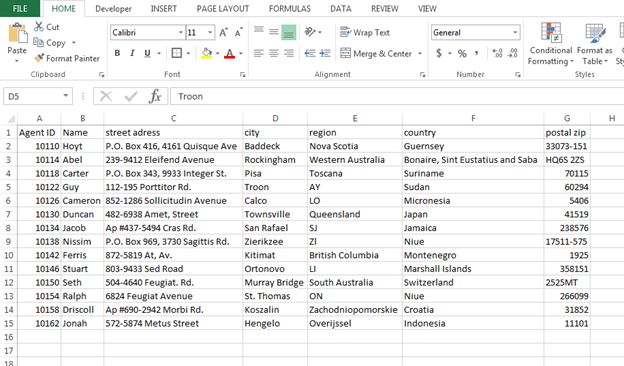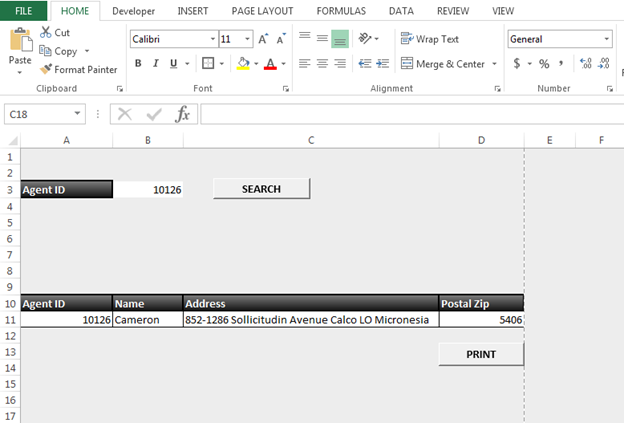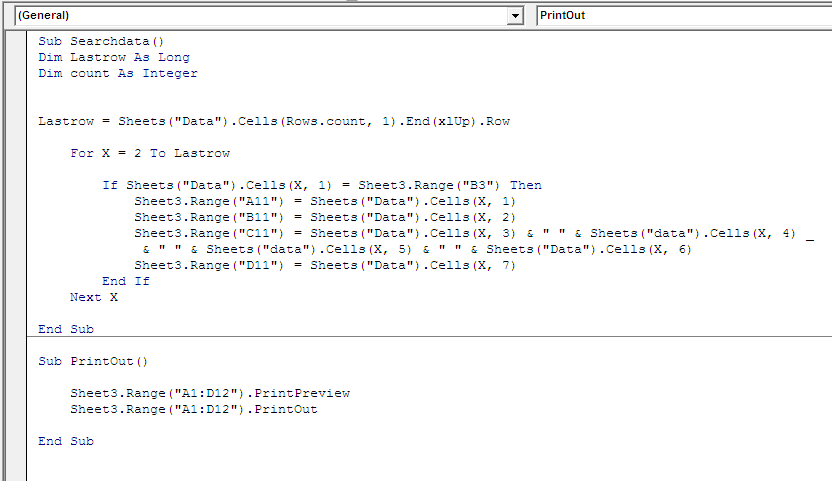How to Create a Form for Search and Print through VBA in Microsoft Excel

In this article, we are going to learn how to create a user form for search and print through VBA in Microsoft Excel.

Let’s understand with a simple exercise:-

We have data in Sheet 1 in which column A contains Agent Id, column B contains Name, Column C Address, column D city, column E region, column F country and column G contains postal zip.In the next sheet (sheet 2), we want to retrieve the details according to the below-mentioned image:-If we search the Agent Id, then all details should appear in the range A11:D11. And then give the printout.

Follow below given steps to Search and Print the details:-

• Press the key F11 to open the Visual Basic Editor
• Insert the module to write the code for search button
• Write the below-mentioned code:-

```Sub Searchdata()
Dim Lastrow As Long
Dim count As Integer

Lastrow = Sheets("Data").Cells(Rows.count, 1).End(xlUp).Row

For X = 2 To Lastrow
If Sheets("Data").Cells(X, 1) = Sheet3.Range("B3") Then
Sheet3.Range("A11") = Sheets("Data").Cells(X, 1)
Sheet3.Range("B11") = Sheets("Data").Cells(X, 2)
Sheet3.Range("C11") = Sheets("Data").Cells(X, 3) & " " & Sheets("data").Cells(X, 4) _
& " " & Sheets("data").Cells(X, 5) & " " & Sheets("Data").Cells(X, 6)
Sheet3.Range("D11") = Sheets("Data").Cells(X, 7)
End If
Next X
End Sub

```

Code Explanation:- First, we will define the variables and then we have defined which row will be the last row, and then we have defined what and where the Agent Id will be searched and then how the range will be updated for the searched Agent ID.

Now, we will assign this macro to the Search button.

• Right click on the button
• Click on Assign Macro
• Here, our macro subject is Searchdata, click on searchdata and then click on OK

Run the Macro:-

• To run the macro, click on Search button
• If the Agent Id will be there then details will get updated otherwise details will not appear

To give the print out, assign the below-mentioned macro:-

• Insert the button from Developer tab>Insert>Button(form control)
• Write the macro in the same model where we have written the macro for Searchdata, with the subject name of Printout

```Sub PrintOut()
Sheet3.Range("A1:D12").PrintPreview
Sheet3.Range("A1:D12").PrintOut
End Sub
```

• Assign the macro to button as we have assigned to searchdataIn this way, we can search the data and then can give the printout to the searched details through VBA in Microsoft Excel.